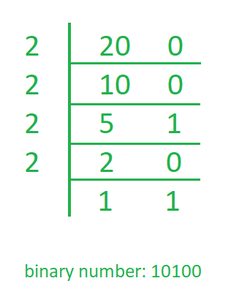Open in App
Not now

# Print Binary Equivalent of an Integer using Recursion in Java

• Difficulty Level : Medium
• Last Updated : 18 Sep, 2022

Given an integer number as input, we need to write a program to convert the given Integer number into an equivalent binary number by using JAVA. BigInteger class Is used for the mathematical operation which involves very big integer calculations that are outside the limit of all available primitive data types.

Examples:

```Input : 1
Output:    1

Input : 12
Output: 1100

Input : 32
Output: 100000```

Algorithm

1. Keep the track of remainder when the number is divided by 2 in an array.
2. Divide the number by 2.
3. Repeat the above two steps until the number is greater than zero.
4. Print the array in reverse order now.

Step-wise Execution: Suppose the binary number is 20.

• Remainder, when 20 is divided by 2, is zero. Therefore, a = 0.
• Divide 20 by 2. New number is 20/2 = 10.
• The remainder, when 10 is divided by 2, is zero. Therefore, a = 0.
• Divide 10 by 2. New number is 10/2 = 5.
• The remainder, when 5 is divided by 2, is 1. Therefore, a = 1.
• Divide 5 by 2. New number is 5/2 = 2.
• The remainder, when 2 is divided by 2, is zero. Therefore, a = 0.
• Divide 2 by 2. New number is 2/2 = 1.
• The remainder, when 1 is divided by 2, is 1. Therefore, a = 1.
• Divide 1 by 2. New number is 1/2 = 0.
• Since number becomes = 0. Print the array in reverse order. Therefore, the equivalent binary number is 10100.

The below image shows it more clearly, the process.Below is the implementation of the above idea in JAVA.

## Java

 `// Java Program to Print Binary``// Equivalent of an Integer``// using Recursion``import` `java.util.*;``class` `GFG {` `    ``public` `static` `int` `binaryConv(``int` `n)``    ``{``        ``if` `(n == ``1``) {` `            ``return` `1``;``        ``}``        ``return` `binaryConv(n / ``2``) * ``10` `+ n % ``2``;``    ``}``    ``public` `static` `void` `main(String[] args)``    ``{``        ``int` `N = ``20``;``        ``System.out.println(binaryConv(N));``    ``}``}`

Output

`10100`

Time Complexity: O (log(n))

Auxiliary Space: O(logn) for call stack

B. Conversion using BigInteger class

## Java

 `// Java Program to Print Binary``// Equivalent of an Integer``// using Recursion``import` `java.util.*;``import` `java.math.*;``class` `GFG {` `    ``public` `static` `BigInteger binaryConv(BigInteger n)``    ``{``        ``if` `(n.compareTo(BigInteger.valueOf(``1``)) == ``0``) {` `            ``return` `BigInteger.valueOf(``1``);``        ``}``        ``return` `((binaryConv(n.divide(BigInteger.valueOf(``2``))).multiply(BigInteger.valueOf(``10``))).add(n.mod(BigInteger.valueOf(``2``))));``    ``}``    ``public` `static` `void` `main(String[] args)``    ``{``        ``BigInteger N = ``new` `BigInteger(``"9876543210987543210"``);``        ``System.out.println(binaryConv(N));``    ``}``}`

Output

`1000100100010000100001111011100011100011101101010101101010101010`

Time Complexity: O (log(n))

My Personal Notes arrow_drop_up